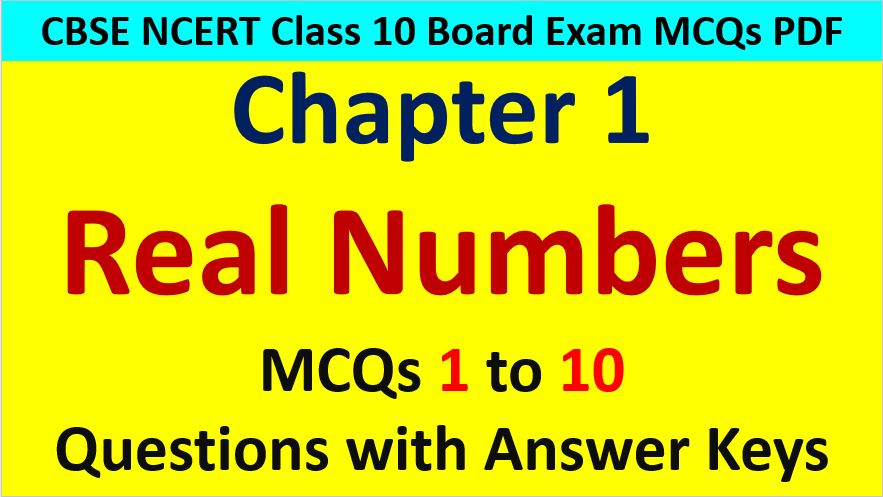Friday, October 22, 2021
Home > Latest Announcement > MCQ Questions for Class 10 Maths Chapter 1 Real Numbers with Answer Keys PDF

# MCQ Questions for Class 10 Maths Chapter 1 Real Numbers with Answer Keys PDFHi students, Welcome to AMBiPi (Amans Maths Blogs). In this article, you will get MCQ Questions for Class 10 Maths Chapter 1 Real Numbers with Answer Keys PDF. You can download this PDF and save it in your mobile device or laptop etc.

MCQ Questions for Class 10 Maths Chapter 1 Real Numbers Question No 1:

The decimal expansion of number 441 / (22 x 53 x 7) has

Option A : a terminating decimal

Option B : non-terminating but repeating

Option C : non-terminating and non-repeating

Option D : terminating after two places of decimals

Option A : a terminating decimal

MCQ Questions for Class 10 Maths Chapter 1 Real Numbers Question No 2:

The decimal form of 129 / (22 x 57 x 75) has

Option A : a terminating decimal

Option B : non-terminating but repeating

Option C : non-terminating and non-repeating

Option D : terminating after two places of decimals

Option C : non-terminating and non-repeating

MCQ Questions for Class 10 Maths Chapter 1 Real Numbers Question No 3:

The decimal expansion of the rational number 33 / (22 x 5) will terminate after

Option A : one decimal place

Option B : two decimal places

Option C : three decimal places

Option D : more than three decimal places

Option B : two decimal places

MCQ Questions for Class 10 Maths Chapter 1 Real Numbers Question No 4:

HCF of 8, 9, 25 is

Option A : 8

Option B : 9

Option C : 25

Option D : 1

Option D : 1

MCQ Questions for Class 10 Maths Chapter 1 Real Numbers Question No 5:

The decimal expansion of 22/7 is

Option A : a terminating decimal

Option B : non-terminating but repeating

Option C : non-terminating and non-repeating

Option D : terminating after two places of decimals

Option B : non-terminating but repeating

MCQ Questions for Class 10 Maths Chapter 1 Real Numbers Question No 6:

Which of the following is not irrational?

Option A : (2 – √3)/2

Option B : (√2 + √3)/2

Option C : (√2 -√3)(√2 + √3)

Option D : 2√7/3

Option A : a terminating decimal

MCQ Questions for Class 10 Maths Chapter 1 Real Numbers Question No 7:

The product of a rational and irrational number is

Option A : rational

Option B : irrational

Option C : both of above

Option D : none of above

Option B : irrational

MCQ Questions for Class 10 Maths Chapter 1 Real Numbers Question No 8:

The sum of a rational and irrational number is

Option A : rational

Option B : irrational

Option C : both of above

Option D : none of above

Option B : irrational

MCQ Questions for Class 10 Maths Chapter 1 Real Numbers Question No 9:

The largest number that will divide 398, 436 and 542 leaving remainders 7,11 and 15 respectively is

Option A : 17

Option B : 11

Option C : 34

Option D : 45

Option A : 17

MCQ Questions for Class 10 Maths Chapter 1 Real Numbers Question No 10:

A number when divided by 61 gives 27 as a quotient and 32 as a remainder. Find the number.

Option A : 1967

Option B : 1796

Option C : 1679

Option D : 1569